# How to do Deep Learning on Graphs with Graph Convolutional NetworksMachine learning on graphs is a difficult task due to the highly complex, but also informative graph structure. This post is the second in a series on how to do deep learning on graphs with Graph Convolutional Networks (GCNs), a powerful type of neural network designed to work directly on graphs and leverage their structural information. I will provide a brief recap of the previous post, but you can find the other parts of the series here:

Machine learning on graphs is a difficult task due to the highly complex, but also informative graph structure. This post is the second in a series on how to do deep learning on graphs with Graph Convolutional Networks (GCNs), a powerful type of neural network designed to work directly on graphs and leverage their structural information. I will provide a brief recap of the previous post, but you can find the other parts of the series here:

1. A High-Level Introduction to Graph Convolutional Networks
2. Semi-Supervised Learning with Spectral Graph Convolutions (this)

### A Brief Recap

In my previous post on GCNs, we a saw a simple mathematical framework for expressing propagation in GCNs. In short, given an N × F⁰ feature matrix X and a matrix representation of the graph structure, e.g., the N × N adjacency matrix A of G, each hidden layer in the GCN can be expressed as Hⁱ = f(Hⁱ⁻¹, A)) where H⁰ = X and f is a propagation rule. Each layer Hⁱ corresponds to an N × F feature matrix where each row is a feature representation of a node.

We saw propagation rules of the form

1. f(Hⁱ, A) = σ(AHⁱWⁱ), and
2. f(Hⁱ, A) = σ(D⁻¹ÂHⁱWⁱ) where Â = A + I, I is the identity matrix, and D⁻¹ is the degree matrix of Â.

These rules computes the feature representation of a node as an aggregate of the feature representations of its neighbors before it is transformed by applying the weights Wⁱ and activation function σ. We can make the aggregation and transformation steps more explicit by expressing propagation rules 1 and 2 above as f(Hⁱ, A) = transform(aggregate(A,Hⁱ), Wⁱ) where transform(M, Wⁱ) = σ(MWⁱ) and aggregate(A,Hⁱ) = AHⁱ for rule 1 and aggregate(A,Hⁱ) = D⁻¹Â Hⁱ for rule 2.

As we discussed in the last post, aggregation in rule 1 represents a node as sum of its neighbors feature representations which has two significant shortcomings:

• the aggregated representation of a node does not include its own features, and
• nodes with large degrees will have large values in their feature representation while nodes with small degrees will have small values, which makes can lead to issues with exploding gradients and make it harder to train using algorithms such as stochastic gradient descent which are sensitive to feature scaling.

To fix these two issues, rule 2 first enforces self loops by adding the identity matrix to A and aggregate on using the transformed adjacency matrix Â = A + I. Next, the feature representations are normalized by multiplication with the inverse degree matrix D⁻¹, turning the aggregate into a mean where the scale of the aggregated feature representation is invariant to node degree.

In the following I will refer to rule 1 as the sum rule and rule 2 as the mean rule.

### Spectral Graph Convolutions

A recent paper by Kipf and Welling proposes fast approximate spectral graph convolutions using a spectral propagation rule :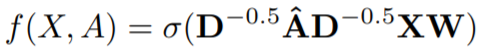Compared to the sum and mean rules discussed in the previous post, the spectral rule differs only in the choice of aggregate function. Although it is somewhat similar to the mean rule in that it normalizes the aggregate using the degree matrix D raised to a negative power, the normalization is asymmetric. Let’s try it out and see what it does.

#### Aggregation as a Weighted Sum

We can understand the aggregation functions I’ve presented thus far as weighted sums where each aggregation rule differ only in their choice of weights. We’ll first see how we can express the relatively simple sum and mean rules as weighted sums before moving on to the spectral rule.

The Sum Rule

To see how the aggregate feature representation of the ith node is computed using the sum rule, we see how the ith row in the aggregate is computed.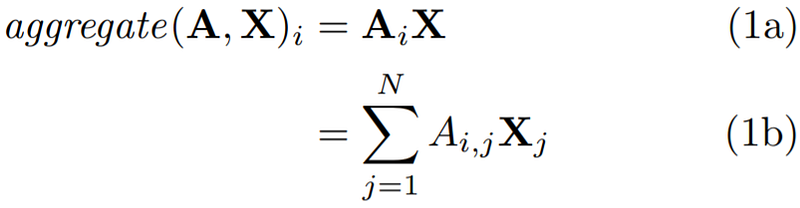The Sum Rule as a Weighted Sum

As shown above in Equation 1a, we can compute the aggregate feature representation of the ith node as a vector-matrix product. We can formulate this vector-matrix product as a simple weighted sum, as shown in Equation 1b, where we sum over each of the N rows in X.

The contribution of the jth node in the aggregate in Equation 1b is determined by the value of the jth column of the ith row of A. Since A is an adjacency matrix, this value is 1 if the jth node is a neighbor of the ith node, and is otherwise 0. Thus, Equation 1b corresponds to summing up the feature representations of the neighbors of the ith node. This confirms the informal observations from the previous post.

In conclusion, the contribution of each neighbor depends solely on the neighborhood defined by the adjacency matrix A.

The Mean Rule

To see how the mean rule aggregates node representations, we again see how the ith row in the aggregate is computed, now using the mean rule. For simplicity, we only consider the mean rule on the “raw“ adjacency matrix without addition between A and the identity matrix I which simply corresponds to adding self-loops to the graph.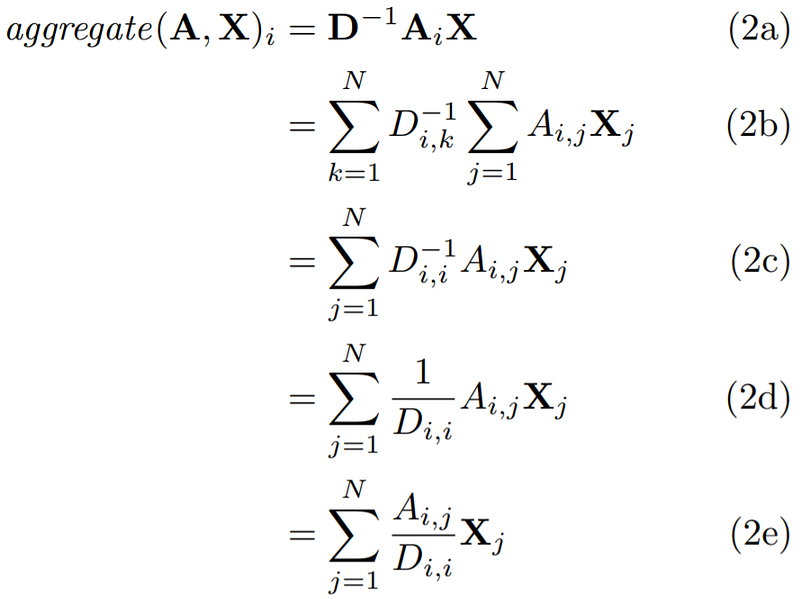The Mean Rule as a Weighted Sum

As seen in the equations above, the derivation is now slightly longer. In Equation 2a we now first transform the adjacency matrix A by multiplying it with the inverse degree matrix D. This computation is made more explicit in Equation 2b. The inverse degree matrix is a diagional matrix where the values along the diagonal are inverse node degrees s.t. the value at position (i, i) is the inverse degree of the ith node. Thus, we can remove one of the summation signs yielding Equation 2c. Equation 2c can be further reduced yieding Equations 2d and 2e.

As shown by Equation 2e, we now again sum over each of the N rows in the adjacency matrix A. As mentioned during the discussion of the sum rule, this corresponds to summing over each the ith node’s neighbors. However, the weights in the weighted sum in Equation 2e are now guaranteed to sum to 1 by with the degree of the ith node. Thus, Equation 2e corresponds to a mean over the feature representations of the neighbors of the ith node.

Whereas the sum rule depends solely on the neighborhood defined by the adjacency matrix A, the mean rule also depends on node degrees.

The Spectral Rule

We now have a useful framework in place to analyse the spectral rule. Let’s see where it takes us!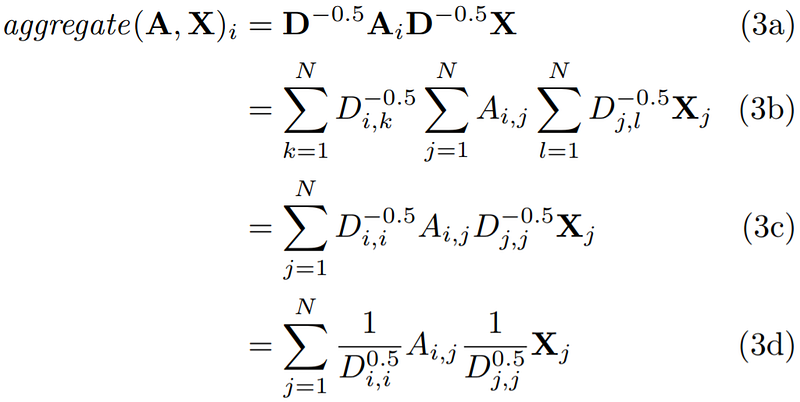The Spectral Rule as a Weighted Sum

As with the mean rule, we transform the adjacency matrix A using the degree matrix D. However, as shown in Equation 3a, we raise the degree matrix to the power of -0.5 and multiply it on each side of A. This operation can be broken down as shown in Equation 3b. Recall again, that degree matrices (and powers thereof) are diagonal. We can therefore simplify Equation 3b further, until we reach the expression in Equation 3e.

Equation 3e shows something quite interesting. When computing the aggregate feature representation of the ith node, we not only take into consideration the degree of the ith node, but also the degree of the jth node.

Similar to the mean rule, the spectral rule normalizes the aggregate s.t. the aggregate feature representation remains roughly on the same scale as the input features. However, the spectral rule weighs neighbor in the weighted sum higher if they have a low-degree and lower if they have a high-degree. This may be useful when low-degree neighbors provide more useful information than high-degree neighbors.

### Semi-Supervised Classification with GCNs

In addition to the spectral rule, Kipf and Welling demonstrate how GCNs can be used for semi-supervised classification . So far we have implicitly assumed that the entire graph is available, i.e., that we are in a transductive setting. In other words, we know all the nodes, but not all the node labels.

In all the rules we’ve seen, we aggregate over node neighborhoods, and thus nodes that share neighbors tend to have similar feature representations. This property is very useful if the graph exhibits homophily, i.e., that connected nodes tend to be similar (e.g. have the same label). Homophily occurs in many real networks, and particularly social networks exhibit strong homophily.

As we saw in the previous post, even a randomly initialized GCN can achieve good separation between the feature representations of nodes in a homophilic graph just by using the graph structure. We can take this a step further by training the GCN on the labeled nodes, effectively propagating the node label information to unlabelled nodes. This can be done as follows :

1. Perform forward propagation through the GCN.
2. Apply the sigmoid function row-wise on the last layer in the GCN.
3. Compute the cross entropy loss on known node labels.
4. Backpropagate the loss and update the weight matrices W in each layer.

### Community Prediction in Zachary’s Karate Club

Let’s see how the spectral rule propagates node label information to unlabelled nodes using semi-supervised learning. As in the previous post, we will use Zachary’s Karate Club as an example.

#### Zachary’s Karate Club

Briefly, Zachary’s Karate Club is a small social network where a conflict arises between the administrator and instructor in a karate club. The task is to predict which side of the conflict each member of the karate club chooses. The graph representation of the network can be seen below. Each node represents a member of the karate club and a link between members indicate that they interact outside the club. The Administrator and Instructor marked with A and I, respectively.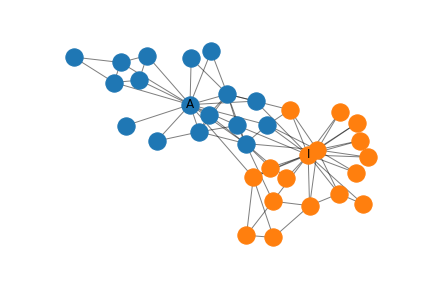Zachary’s Karate Club

#### Spectral Graph Convolutions in MXNet

I implement the spectral rule in MXNet, an easy-to-use and efficient deep learning framework. The implementation is as follows:

```class SpectralRule(HybridBlock):
def __init__(self,
A, in_units, out_units,
activation, **kwargs):
super().__init__(**kwargs)
I = nd.eye(*A.shape)
A_hat = A.copy() + I
D = nd.sum(A_hat, axis=0)
D_inv = D**-0.5
D_inv = nd.diag(D_inv)
A_hat = D_inv * A_hat * D_inv

```    self.in_units, self.out_units = in_units, out_units

with self.name_scope():
self.A_hat = self.params.get_constant('A_hat', A_hat)
self.W = self.params.get(
'W', shape=(self.in_units, self.out_units)
)
if activation == 'ident':
self.activation = lambda X: X
else:
self.activation = Activation(activation)
def hybrid_forward(self, F, X, A_hat, W):
aggregate = F.dot(A_hat, X)
propagate = self.activation(
F.dot(aggregate, W))
return propagate```
```

init takes as input an adjacency matrix A along with the input and output dimensionality of each node’s feature representation from the graph convolutional layer;in_units, and out_units, respectively. Self-loops are added to the adjacency matrix A through addition with the identity matrix I, calculate the degree matrix D, and transform the adjacency matrix A to A_hat as specified by the spectral rule. This transformation is not strictly necessary, but is more computationally efficient since the transformation would otherwise be performed during each forward pass of the layer.

Finally, in the with clause in init, we store two model parameters — A_hat is stored as a constant and the weight matrix W is stored as a trainable parameter.

hybrid_forward is where the magic happens. In the forward pass we execute this method with the following inputs: X, the output of the previous layer, and the parameters A_hat and W that we defined in the constructor init.

#### Building the Graph Convolutional Network

Now that we have an implementation of the spectral rule, we can stack such layers on top of each other . We use a two-layer architecture similar to the one in the previous post, where the first hidden layer has 4 units and the second hidden layer has 2 units. This architecture makes it easy visualize the resulting 2-dimensional embeddings. It differs from the architecture in the previous post in three ways:

• We use the spectral rule rather than the mean rule.
• We use different activation functions: the tanh activation function is used in the first layer since the probability of dead neurons would otherwise be quite high and the second layer uses the identity function since we use the last layer to classify nodes.

Finally, we add a logistic regression layer on top of the GCN for node classification.

The Python implementation of the above architecture is as follows.

```def build_model(A, X):
model = HybridSequential()
with model.name_scope():
features = build_features(A, X)
classifier = LogisticRegressor()
model.initialize(Uniform(1))
return model, features
```

I have separated the feature learning part of the network that contains the graph convolutional layers into a features component and the classification part into the classifier component. The separate features component makes it easier to visualise the activations of these layers later. The LogisticRegressor used as the classifier is a classification layer that performs logistic regression by summing over the features of each node provided by the last graph convolutional layer and applying the sigmoid function on this sum.

For completeness, the code to construct the features component is

```def build_features(A, X):
hidden_layer_specs = [(4, 'tanh'), (2, 'tanh')]
in_units = in_units=X.shape
```features = HybridSequential()
with features.name_scope():
for layer_size, activation_func in hidden_layer_specs:
layer = SpectralRule(
A, in_units=in_units, out_units=layer_size,
activation=activation_func)
in_units = layer_size
return features```
```

and the code for the LogisticRegressor is

```class LogisticRegressor(HybridBlock):
def init(self, in_units, kwargs):
super().init(kwargs)
with self.name_scope():
self.w = self.params.get(
'w', shape=(1, in_units)
)
self.b = self.params.get(
'b', shape=(1, 1)
)
def hybrid_forward(self, F, X, w, b):
# Change shape of b to comply with MXnet addition API
b = F.broadcast_axis(b, axis=(0,1), size=(34, 1))
y = F.dot(X, w, transpose_b=True) + b
return F.sigmoid(y)
```

#### Training the GCN

The code for training the GCN model can be seen below. In brief, I initialize a binary cross entropy loss function, cross_entropy, and an SGD optimizer, trainer to learn the network parameters. Then the model is trained for a a specified number of epochs where the loss is calculated for each training example and the error is backpropagated using loss.backward(). trainer.step is then invoked to update the model parameters. After each epoch, the feature representation constructed by the GCN layer is stored in the feature_representations list which we shall inspect shortly.

```def train(model, features, X, X_train, y_train, epochs):
cross_entropy = SigmoidBinaryCrossEntropyLoss(from_sigmoid=True)
trainer = Trainer(
model.collect_params(), 'sgd',
{'learning_rate': 0.001, 'momentum': 1})
feature_representations = [features(X).asnumpy()]
for e in range(1, epochs + 1):
for i, x in enumerate(X_train):
y = array(y_train)[i]
preds = model(X)[x]
loss = cross_entropy(preds, y)
loss.backward()
trainer.step(1)
feature_representations.append(features(X).asnumpy())
return feature_representations
```

Crucially, only the labels of the instructor and administrator are labeled and the remaining nodes in the network are known, but unlabeled! The GCN can find representations for both labeled and unlabeled nodes during graph convolution and can leverage both sources of information during training to perform semi-supervised learning.

#### Visualizing the Features

As mentioned above, the feature representations at each epoch are stored which allows us to see how the feature representations changes during training. In the following I consider two input feature representations.

Representation 1

In the first representation, we simply use the sparse 34 × 34 identity matrix, I, as the feature matrix X. This representation has the advantage that it can be used in any graphs, but results in an input parameter for each node in the network which requires a substantial amount of memory and computional power for training on large networks and may result in overfitting. Thankfully, the karate club network is quite small. The network is trained for 5000 epochs using this representation.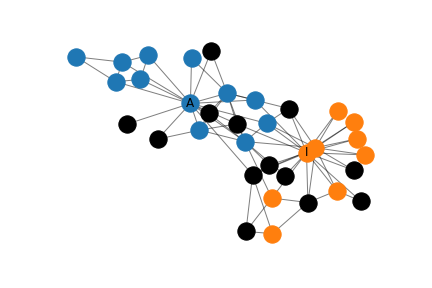Classification Errors in the Karate Club using Representation 1

By collectively classifying all nodes in the network, we get the distribution of errors in the network shown on above. Here, black indicates misclassification. Although a nearly half (41%) of the nodes are misclassified, the nodes that are closely connected to either the administrator or instructor (but not both!) tend to be correctly classified.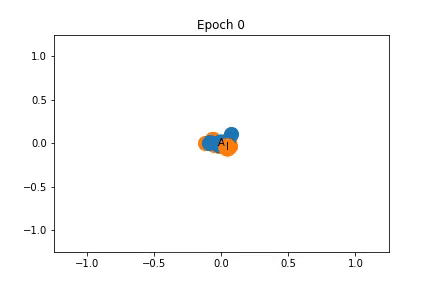Changes in Feature Representation during Training using Representation 1

To the left, I have illustrated how the feature representation changes during training. The nodes are initially closely clustered, but as training progresses the instructor and administrator are pulled apart, dragging some nodes with them.

Although the administrator and instructor are given quite different representations, the nodes they drag with them do not necessarily belong to their community. This is because the graph convolutions embed nodes that share neighbors closely together in the feature space, but two nodes that share neighbors may not be equally connected to the administrator and instructor. In particular, using the identity matrix as the feature matrix results in highly local representations of each node, i.e., nodes that belong to the same area of the graph are likely to be embedded closely together. This makes it difficult for the network to share common knowledge between distant areas in an inductive fashion.

Representation 2

We will improve representation 1 by adding two features that are not specific to any node or area of the network, but measures the connectedness to the administrator and instructor. To this end, we compute the shortest path distance from each node in the network to both the administrator and instructor and concatenate these two features to the previous representation.

On might perhaps consider this cheating a little bit, since we inject global information about the location of each node in the graph; information which should (ideally) be captured by the graph convolutional layers in the features component. However, the graph convolutional layers always have a local perspective and has a limited capacity to capture such information. Still, it serves as a useful tool for understanding GCNs.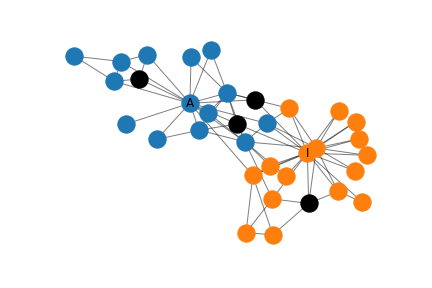Classification Errors in the Karate Club using Representation 1

As before, we collectively classify all nodes in the network and plot the distribution of errors in the network shown on above. This time, only four nodes are misclassified; a significant improvement over representation 1! Upon closer inspection of the feature matrix, these nodes are either equidistant (in a shortest path sense) to the instructor and administrator or are closer to the administrator but belong in the instructor community. The GCN is trained for 250 epochs using representation 1.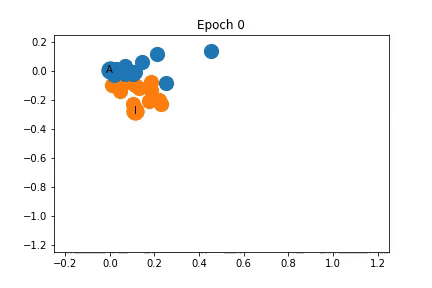Changes in Feature Representation during Training using Representation 2

As shown on the left, the nodes are again clustered quite closely together initially, but are somewhat separated into communities before training even begins! As training progresses the distance between the communities increases.

### What’s Next?

In this post, I have given an in-depth explanation on how aggregation in GCNs is performed and shown how it can be expressed as a weighted sum, using the mean, sum, and spectral rules as examples. My sincere hope is that you will find this framework useful to consider which weights you might want during aggregation in your own graph convolutional network.

I have also shown how to implement and train a GCN in MXNet to perform semi-supervised classification on graphs using spectral graph convolutions with Zachary’s Karate Club as a simple example network. We saw how just using two labeled nodes, it was still possible for the GCN to achieve a high degree of separation between the two network communities in the representation space.

Although there is much more to learn about graph convolutional networks which I hope to have the time to share with you in the future, this is (for now) the final post in the series. If you are interested in further reading, I would like to conclude with the following papers which I have found quite interesting:

1. Inductive Representation Learning on Large Graphs
2. In this paper, Hamilton et al., propose several new aggregate functions that, e.g., uses max/mean pooling or multi-layer perceptrons. In addition, they also propose a simple method to do mini-batch training for GCNs, greatly improving training speed.
3. FastGCN: Fast Learning with Graph Convolutional Networks via Importance Sampling
4. A drawback of the mini-batch method proposed by Hamilton et al., is that the number of nodes in a batch grows exponentially in the number of aggregates performed due to their recursive. Chen et. al, propose their FastGCN method which addresses this shortcoming by performing batched training of graph convolutional layers independently.
5. N-GCN: Multi-scale Graph Convolution for Semi-supervised Node Classification
6. Where FastGCN addresses the problems of training recursive graph convolutional networks, N-GCN challenges the premise that GCNs need to be recursive at all! Abu-El-Haija et al. instead propose a flat architecture with multiple (N) GCNs whose outputs are concatenated together. Each GCN captures the neighborhood at different distances (based on random walk semantics), thus avoiding recursive aggregation. Thanks to Binny Mathew for bringing this to my attention.

## Why You Should Learn R — Learn Data Science with Dataquest

Why should you learn R programming when you're aiming to learn data science? Here are six reasons why R is the right language for you.

## Learn Data Science Today - Data Science Tutorial for Beginners 2020!

How and why to start Learning to be a data scientist in 2020! This Data Science Course will give you a Step by Step idea about the Data Science Career, Data science Hands-On Projects, roles & salary offered to a Data Scientist!

## Exploratory Data Analysis is a significant part of Data Science

Data science is omnipresent to advanced statistical and machine learning methods. For whatever length of time that there is data to analyse, the need to investigate is obvious.

## Learning Data Science through competitions

What are the pros and cons of taking Competitive Data Science as a learning path. What is the best way to learn Data Science and Machine Learning?

## Deep Learning — not only for the big ones

How you can use Deep Learning even for small datasets. When you’re working on Deep Learning algorithms you almost always require a large volume of data to train your model on.